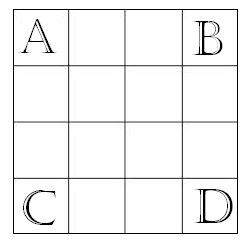# Magic Squares

Logic Level 3A magic square of order 4 is created by putting the integers 1 to 16 into a 4 by 4 square grid so that the sum of the numbers in each row, column and main diagonal is the same. What is the sum of the integers at the corners of this magic square (labeled by letters as shown above)?

×